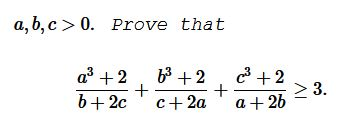# An Inequality from a Vietnamese Problem Book

### Problem### Solution 1

By the AM-GM inequality, $a^3+2\ge 3a,\,$ $b^3+2\ge 3b,\,$ $c^3+2\ge 3c.\,$ Suffice it to show that

$\displaystyle \frac{a}{b+2c}+\frac{b}{c+2a}+\frac{c}{a+2b}\ge 1.$

Let $\displaystyle \frac{a}{b+2c}=x,\,$ $\displaystyle \frac{b}{c+2a}=y,\,$ $\displaystyle \frac{c}{a+2b}=z.\,$ Then $x,y,z\gt 0\,$ and

$\left(\begin{array}{ccc}\,-1&x&2x\\2y&-1&y\\z&2z&-1\end{array}\right)\left(\begin{array}{c}\,a\\b\\c\end{array}\right)=\left(\begin{array}{c}\,0\\0\\0\end{array}\right).$

If the matrix here is invertible, the only solution that the system may have is trivial, but, by the definition, the system has a non-trivial solution $(a\,b\,c)^t,\,$ implying that the determinant of the matrix vanishes:

$\left|\begin{array}{ccc}\,-1&x&2x\\2y&-1&y\\z&2z&-1\end{array}\right|=0.$

It follows that $2(xy+yz+zx)+9xyz=1.\,$ Le $x+y+z=t.\,$ Thne $\displaystyle \frac{2t^2}{3}\ge 2(xy+yz+zx)\,$ and $\displaystyle \frac{t^3}{3}\ge 9xyz,\,$ such that $t^3+2t^2\ge 3,\,$ i.e., $(t-1)(t^2+3t+3)\ge 0,\,$ so that $t\ge 1.\,$ This completes the proof.

### Solution 2

Using Bergstrom's inequality,

\displaystyle \begin{align} \sum_{cycl}\frac{a^3+2}{b+2c}&=\sum_{cycl}\frac{a^4}{ab+2ac}+2\sum_{cycl}\frac{1}{b+2c}\\ &\ge\frac{(a^2+b^2+c^2)^2}{3(ab+bc+ca)}+\frac{2\cdot 3^2}{3(a+b+c)}\\ &\ge\frac{a^2+b^2+c^2}{3}+\frac{6}{a+b+c}\\ &\ge\frac{(a+b+c)^2}{9}+\frac{3}{a+b+c}+\frac{3}{a+b+c}\\ &\ge 3\sqrt{\frac{(a+b+c)^2}{9}\cdot\frac{3}{a+b+c}\cdot\frac{3}{a+b+c}}\\ &=3, \end{align}

because $a^2+b^2+c^2\ge ab+bc+ca\,$ and $3(a^2+b^2+c^2)\ge (a+b+c)^2.$

### Solution 3

We start with $\displaystyle \sum_{cycl}\frac{a}{b+2c}.\,$ Using Bergstrom's inequality,

$\displaystyle \sum_{cycl}\frac{a}{b+2c}\ge\frac\left(\sum_{cycl}a\right)^2}\sum_{cycl}(ab+2ac)}=\frac\left(\sum_{cycl}a\right)^2}3\sum_{cycl}ab}\ge\frac\left(\sum_{cycl}a\right)^2}3\frac{1}{3}\left(\sum_{cycl}a\right)^2}=1$

because $(a+b+c)^2=(a^2+b^2+c^2)+2(ab+2bc+ca)\ge 3(ab+2bc+ca).$

### Acknowledgment

Leo Giugiuc has kindly mailed me the problem and his solution (Solution 1) that shows once again how useful linear algebra may be in proving inequalities. The problem was originally posted by Imad Zak at the mathematical inequalities facebook group. Solution 2 is by Abdur Rehman Zahid.

### Linear Algebra Tools for Proving Inequalities

$\;\left(\displaystyle\left(\frac{a}{b-c}\right)^2+\left(\frac{b}{c-a}\right)^2+\left(\frac{c}{a-b}\right)^2\ge 2\right)$
• Linear Algebra Tools for Proving Inequalities: Cauchy-Binet Formula $\;\left(\displaystyle\left(\sum_{i=1}^{n}\frac{x_i^2}{a_i}\right)\cdot\left(\sum_{1\le i\lt j\le n}a_ia_j(x_iy_j-x_jy_i)^2\right)\ge \sum_{i=1}^{n}a_iy_i^2\right)$
• An Inequality from Gazeta Matematica, March 2016 (If $a^2+b^2+c^2=3\,$ then $(a+c)(1+b)\le 4)$
• An Inequality from Gazeta Matematica, March 2016 II (If $x^2+y^2+z^2+t^2=1\,$ then $\;(x+z)(y+t)\le 4)$
• An Inequality from Gazeta Matematica, March 2016 III $\;(a^2+b^2+1\ge a+ab+b)$
• An Inequality from Gazeta Matematica, March 2016 IV (If $a^2+b^2+c^2=1\,$ then $a+ac+b\le 2)$
• Problem 3980 from Crux Mathematicorum $\;\left(\displaystyle\sum_{cycl}\frac{a+b}{a-b}\prod_{cycl}\frac{a+b}{a-b}\lt\frac{1}{3}\right)$
• NonSquare Matrix as a Tool for Proving an Inequality $\;\left(2(a + b + c)((a + 2b + 3c) \ge (\sqrt{b(a+b)} + 2\sqrt{c(b+c)} + \sqrt{a(c+a)})^2\right)$
• An Inequality in Parallelogram of Unit Area $\;\left(a^2+b^2+c^2+d^2+ac+bd\ge\sqrt{3}\right)$
• Hadamard's Determinant Inequalities and Applications I $\left((2-a-b-c+abc)^2\le (a^2+2)(b^2+2)(c^2+2)\right)$
• Hadamard's Determinant Inequalities and Applications II $\left((n + a - 1)(a - 1)^{n-1} \le a^n\right)$
•